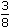# Aptitude - Partnership - Discussion

### Discussion :: Partnership - Data Sufficiency 3 (Q.No.1)

Each of these questions is followed by three statements. You have to study the question and all the three statements given to decide whether any information provided in the statement(s) is redundant and can be dispensed with while answering the given question.

1.

 Three friends, P, Q and R started a partnership business investing money in the ratio of 5 : 4 : 2 respectively for a period of 3 years. What is the amount received by P as his share profit? I. Total amount invested in the business in Rs. 22,000. II. Profit earned at the end of 3 years isof the total investment. III. The average amount of profit earned per year is Rs. 2750.

 [A]. I or II or III [B]. Either III only, or I and II together [C]. Any two of the three [D]. All I, II and III are required. [E]. None of these

Explanation:

 I and II give, profit after 3 years = Rs.3 x 22000= Rs. 8250. 8

From III also, profit after 3 years = Rs. (2750 x 3) = Rs. 8250.P's share = Rs.8250 x 5= Rs. 3750. 11

Thus, (either III is redundant [or] I and II are redundant).Correct answer is (B).

 Praveen said: (Feb 28, 2011) I can't understand how it come 22000.

 Pri said: (Jun 11, 2011) I cant got it.

 Raj Singh said: (Jul 25, 2011) I can't understand how B is correct.

 Karuna said: (Sep 28, 2011) I can't understand how B is correct. Can you give explanation.

 Irfan said: (Jan 18, 2012) Why we multiply by 5/11?

 Yusuf said: (May 29, 2012) Because P's share is 5 out of total 11 (5+4+2). Hence it's multiplied by 5/11.

 Sahil said: (Oct 3, 2015) I believe that the answer is incorrect, if we consider the total investment as x, for option 2, then we can solve the entire question.

 Varun said: (Jul 31, 2017) Take I nd II 22000 of inverstment. 3/8 of profit for yrs == 22000*(3/8) == 8250, for 5:4:2 ==8250 == 3750:3000:1500, Share of P after 3 yrs 13750 also possible.

 Kalyani said: (Jun 11, 2020) I can't understand this. Please anyone explain this in detail.